Ex 10.3

Chapter 10 Class 12 Vector Algebra
Serial order wise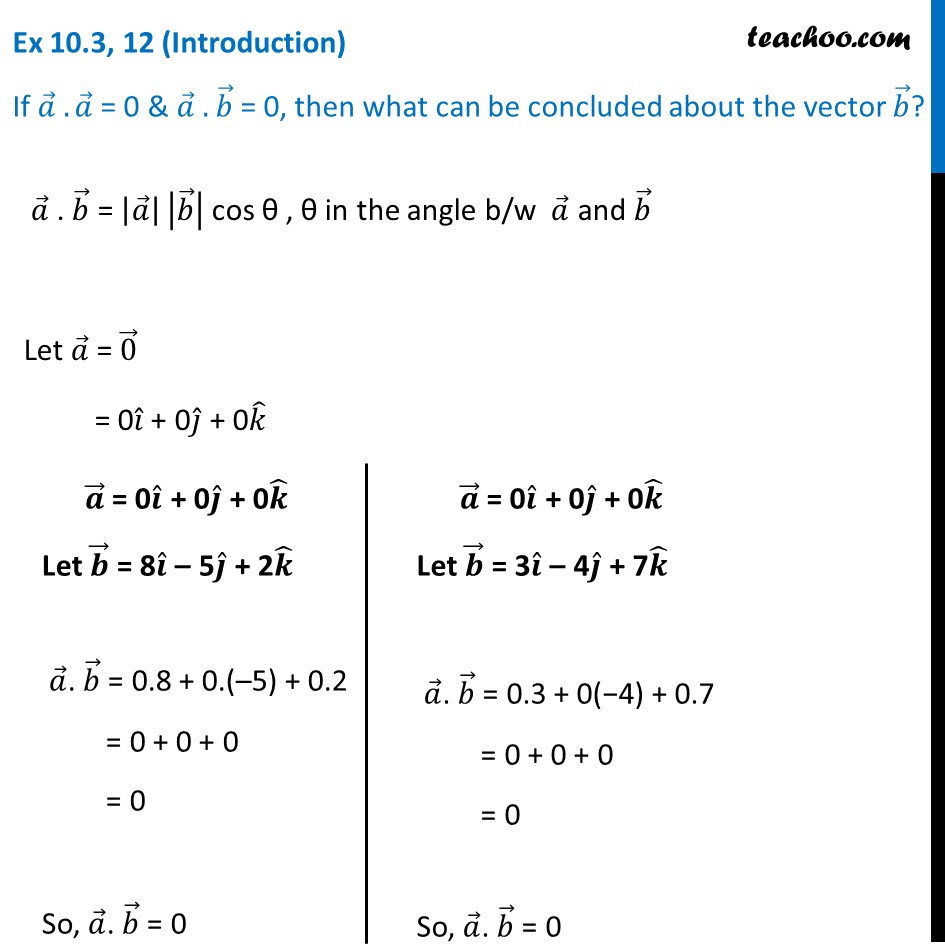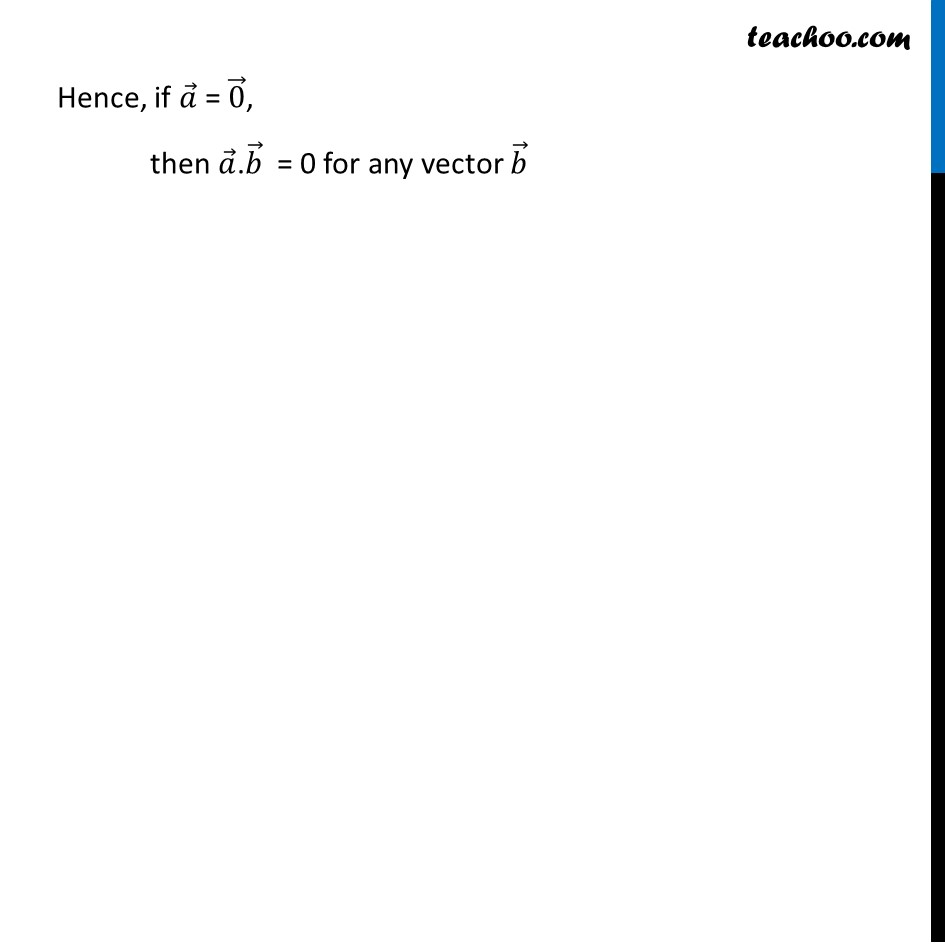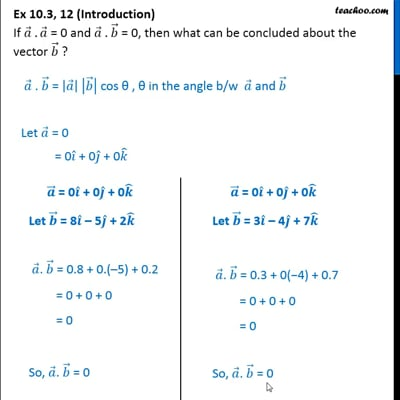This video is only available for Teachoo black users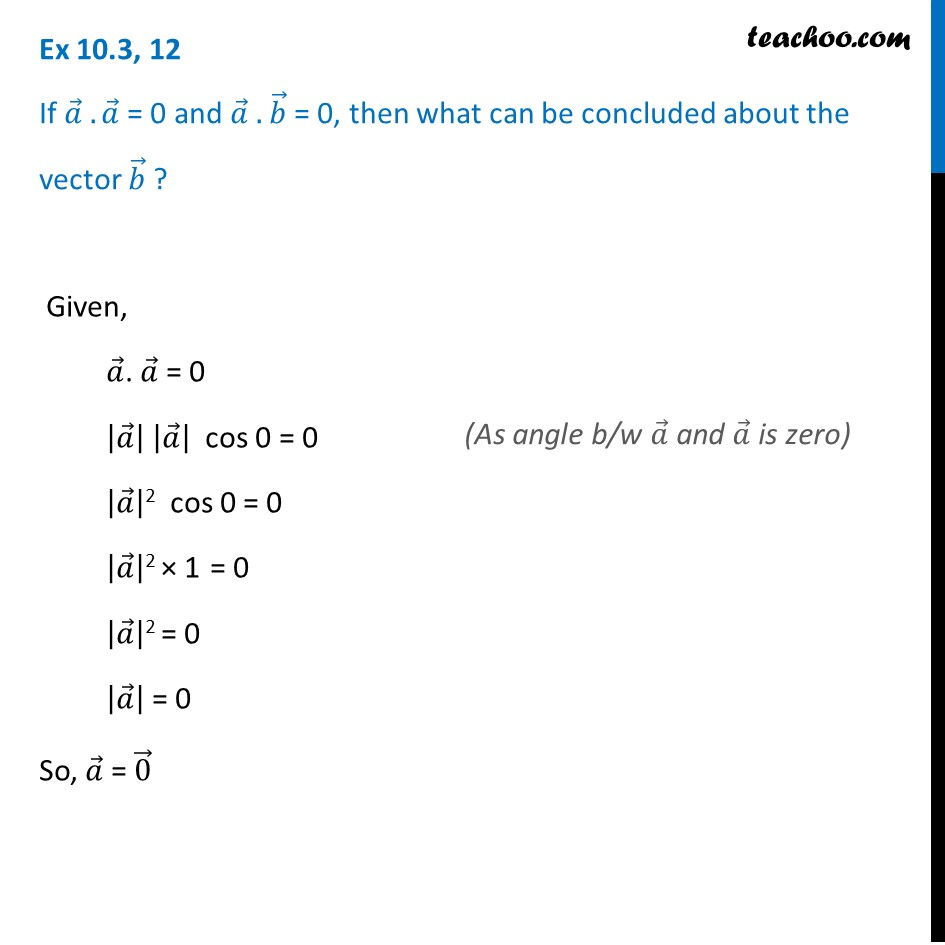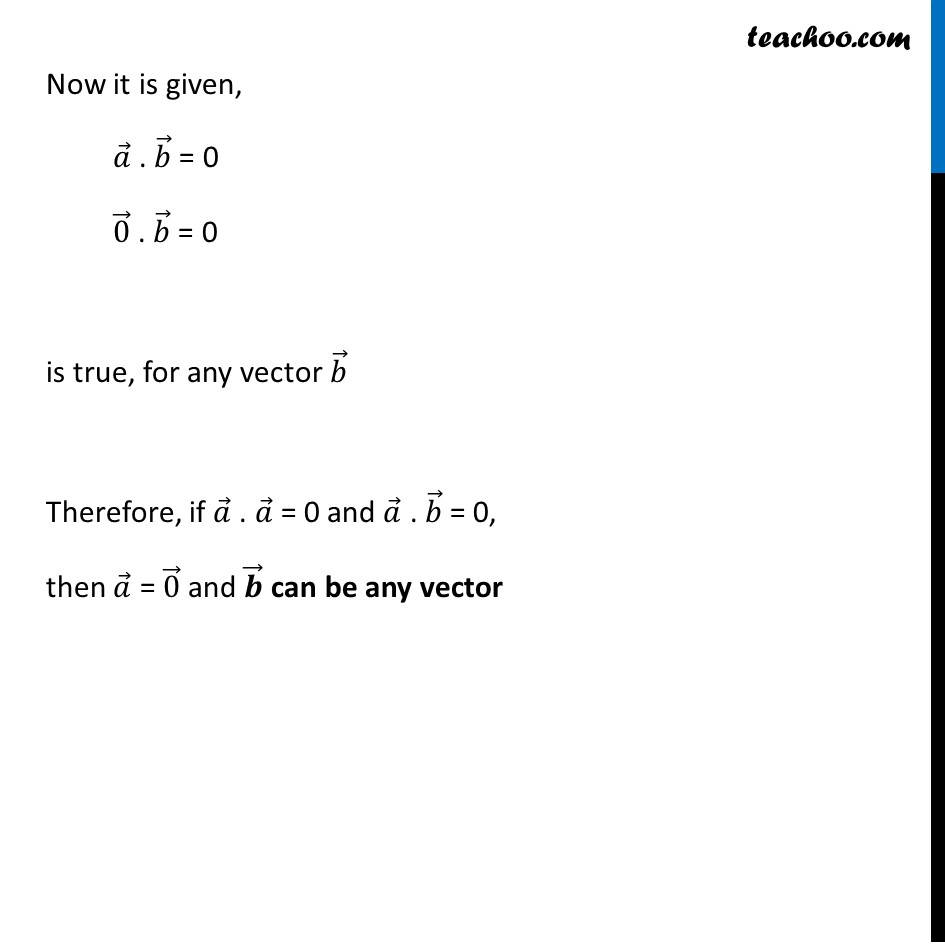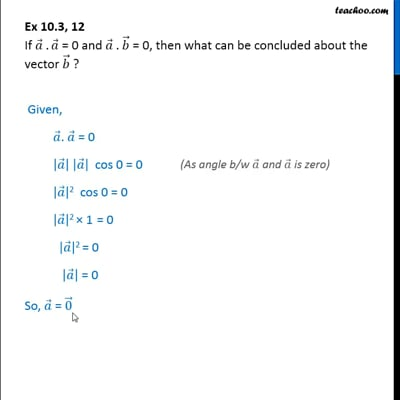This video is only available for Teachoo black users

Solve all your doubts with Teachoo Black (new monthly pack available now!)

### Transcript

Ex 10.3, 12 (Introduction) If 𝑎 ⃗ .𝑎 ⃗ = 0 & 𝑎 ⃗ . 𝑏 ⃗ = 0, then what can be concluded about the vector 𝑏 ⃗? 𝑎 ⃗ . 𝑏 ⃗ = |𝑎 ⃗ | |𝑏 ⃗ | cos θ , θ in the angle b/w 𝑎 ⃗ and 𝑏 ⃗ Let 𝑎 ⃗ = 0 ⃗ = 0𝑖 ̂ + 0𝑗 ̂ + 0𝑘 ̂ 𝒂 ⃗ = 0𝒊 ̂ + 0𝒋 ̂ + 0𝒌 ̂ Let 𝒃 ⃗ = 8𝒊 ̂ – 5𝒋 ̂ + 2𝒌 ̂ 𝑎 ⃗. 𝑏 ⃗ = 0.8 + 0.(–5) + 0.2 = 0 + 0 + 0 = 0 So, 𝑎 ⃗. 𝑏 ⃗ = 0 𝒂 ⃗ = 0𝒊 ̂ + 0𝒋 ̂ + 0𝒌 ̂ Let 𝒃 ⃗ = 3𝒊 ̂ – 4𝒋 ̂ + 7𝒌 ̂ 𝑎 ⃗. 𝑏 ⃗ = 0.3 + 0(−4) + 0.7 = 0 + 0 + 0 = 0 So, 𝑎 ⃗. 𝑏 ⃗ = 0 Hence, if 𝑎 ⃗ = 0 ⃗, then 𝑎 ⃗.𝑏 ⃗ = 0 for any vector 𝑏 ⃗ Ex 10.3, 12 If 𝑎 ⃗ .𝑎 ⃗ = 0 and 𝑎 ⃗ . 𝑏 ⃗ = 0, then what can be concluded about the vector 𝑏 ⃗ ? Given, 𝑎 ⃗. 𝑎 ⃗ = 0 |𝑎 ⃗ | |𝑎 ⃗ | cos 0 = 0 |𝑎 ⃗ |2 cos 0 = 0 |𝑎 ⃗ |2 × 1 = 0 |𝑎 ⃗ |2 = 0 |𝑎 ⃗ | = 0 So, 𝑎 ⃗ = 0 ⃗ Now it is given, 𝑎 ⃗ . 𝑏 ⃗ = 0 0 ⃗ . 𝑏 ⃗ = 0 is true, for any vector 𝑏 ⃗ Therefore, if 𝑎 ⃗ . 𝑎 ⃗ = 0 and 𝑎 ⃗ . 𝑏 ⃗ = 0, then 𝑎 ⃗ = 0 ⃗ and 𝒃 ⃗ can be any vector